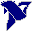# LabVIEW

cancel
Showing results for
Did you mean:

## method to convert the measurement from volts to force ?

I have a PCB impact hammer (ref 086D20) connected to card NI 9234. I want to interpret the voltage measurements (in Volts) to force (in Newton). I tried to multiply the hammer sensibility (0.23mV/N) by the voltage values but it seems that is not correct. For the purpose of using Matlab to write a standalone executable application, I don't want to use others intermediate programs (for exemple NI LabView or LabView Signal Express).

So, I'm looking for a method, more specially an algorithm, to convert the voltage to the force. Someone can help me ?

Thank you

Message 1 of 4
(2,698 Views)

## Re: method to convert the measurement from volts to force ?

Hi newbie,

- how do you read the sensor data? What are the specs for this measurement?

- How do you come to the conclusion your voltage values do seem incorrect?

- How does Matlab come into this topic? Why don't you ask in a Matlab forum when you want to use this IDE?

an algorithm, to convert the voltage to the force

You need to apply all specs given in the sensor's manual…

Or ask PCB as they offer "Total Customer Satisfaction"! 😄

Best regards,
GerdWusing LV2011SP1 + LV2017 (+LV2020 sometimes) on Win10+cRIO
Message 2 of 4
(2,690 Views)

## Re: method to convert the measurement from volts to force ?

Force [N]=(1.0/(0.23*1000)) * Voltage [V]

Agreed in principle this question is nothing to do with LabVIEW.

Message 3 of 4
(2,681 Views)

## Re: method to convert the measurement from volts to force ?

In fact, my question is indirectly related with LabView (especially LabView Signal Express). Before using Matlab to write my application, I used LabView Signal Express to test my system (PCB impact hammer and NI 9234). When I choose the voltage as my analog input signal, I have a curve of voltage according to time. This cuve contains many positive and negative peaks (I did just one shock of hammer). However, when I change the kind of input signal to force (N), i obtain a new curve that contained just one positive peak. This result corresponds well with my test in which there is just one shock of hammer.

Through these 2 tests, I think that must have a method to convert the voltage measurements to force, and that is not simple as multiply the voltage by a constant (sensibility or sensibility^-1). It must be something more complicated. I readed the documents of LabView Signal Express, but I cannot find the method on which this sofware based on to interpret the voltage to real force.

Message 4 of 4
(2,661 Views)